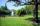High school + Heron's formula - examples

1. TriangleTriangle KLM is given by plane coordinates of vertices: K[-12, -11] L[-15, -18] M[-13, -12]. Calculate its area and itsinterior angles.
2. Triangle ABCCalculate the sides of triangle ABC with area 1404 cm2 and if a: b: c = 12:7:18
3. DescribedCalculate perimeter of the circle described by a triangle with sides 478, 255, 352.
4. TrapezoidCalculate area of trapezoid ABCD with sides |AD|= 57 cm, |DC|=30 cm, |CB|=7 cm, |AB|=40 cm..
5. Triangle SSSCalculate perimeter and area of ​​a triangle ABC, if a=40, b=36 and c=36.
6. Cardboard boxWe want to make a cardboard box shaped quadrangular prism with rhombic base. Rhombus has a side of 5 cm and 8 cm one diagonal long. The height of the box to be 12 cm. The box will be open at the top. How many square centimeters cardboard we need, if we cal
7. BoxCardboard box shaped quadrangular prism with a rhombic base. Rhombus has a side 5 cm and one diagonal 8 cm long and height of the box is 12 cm. The box will open at the top. How many cm2 of cardboard we need to cover overlap and joints that are 5% of ar
8. Heron backlawCalculate missing side in a triangle with sides 17 and 34 and area 275.
9. ParkIn the newly built park will be permanently placed a rotating sprayer irrigation of lawns. Determine the largest radius of the circle which can irrigate by sprayer P so not to spray park visitors on line AB. Distance AB = 55 m, AP = 36 m and BP = 28 m.
10. Circles 2Calculate the area bounded by the circumscribed and inscribed circle in triangle with sides 12 cm, 14 cm, 18 cm.
11. Trapezoid - hard exampleBase of the trapezoid are: 24, 16 cm. Diagonal 22, 26 cm. Calculate its area and perimeter.
12. Area and two anglesCalculate the size of all sides and internal angles of a triangle ABC, if it is given by area S = 501.9; and two internal angles α = 15°28' and β = 45°.
13. SailsWe known heights 220, 165 and 132 of sail. It has triangular shape. What is the surface of the sail?
14. Sides of the triangleCalculate triangle sides where its area is S = 84 cm2 and a = x, b = x + 1, xc = x + 2
15. Four sides of trapezoidTrapezoid is given by length of four sides: 40.5 42.5 52.8 35.0. Calculate its area.
16. TriangleThe triangle has known all three sides: a=5.5 m, b=5.3 m, c= 7.8 m. Calculate area of ​this triangle.
17. TriangleDetermine whether a triangle can be formed with the given side lengths. If so, use Heron's formula to find the area of the triangle. a = 158 b = 185 c = 201
18. The farmerThe farmer would like to first seed his small field. The required amount depends on the seed area. Field has a triangular shape. The farmer had fenced field, so he knows the lengths of the sides: 119, 111 and 90 meters. Find a suitable way to determine th
19. Sides ratioCalculate the circumference of a triangle with area 84 cm2 if a:b:c = 10:17:21
20. Find the areaFind the area of the triangle with the given measurements. Round the solution to the nearest hundredth if necessary. A = 50°, b = 30 ft, c = 14 ft

Do you have an interesting mathematical example that you can't solve it? Enter it, and we can try to solve it.

To this e-mail address, we will reply solution; solved examples are also published here. Please enter e-mail correctly and check whether you don't have a full mailbox.